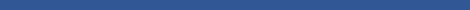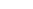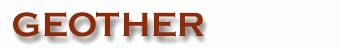Geometric Version 1.0
GEOTHER (GEOmetry THeorem provER), a module of Epsilon, is an environment implemented by Dongming Wang in Maple with drawing routines and interface written previously in C and now in Java for manipulating and proving geometric theorems. In GEOTHER a theorem is specified by means of predicates of the form Theorem(H,C,X) asserting that H implies C, where H and C are lists or sets of predicates that correspond to the geometric hypotheses and the conclusion of the theorem, and the optional X is a list of variables used for internal computation. The information contained in the specification may be all that is needed in order to manipulate and prove the theorem. From the specification, GEOTHER can automatically
• assign coordinates to each point in some optimal manner;
• translate the predicate representation of the theorem into an English or Chinese statement, into a first-order logical formula, or into algebraic expressions;
• draw one or several diagrams for the theorem - the drawn diagrams may be animated and modified with a mouse click and dragging, and saved as PostScript files;
• prove the theorem using any of the five algebraic provers;
• translate the generated algebraic nondegeneracy conditions into geometric/predicate form;
• generate an HTML, LaTeX, and/or PostScript file documenting the theorem and its proof.
The assignment of coordinates to points can be done optionally by the user. The algebraic provers are based on Wu's method, the Gröbner basis method, and a method proposed by D. Wang. GEOTHER can run with a menu-driven graphic user interface and contains a collection of theorems in both elementary and differential geometries with sample specifications that have been proved. These proved theorems include those named after Steiner, Morley, Thébault, MacLane, and Bertrand. The package also has functions for handling the collection of theorems and on-line help for all functions with examples.

Excerpts with modification from Mathematics Mechanization and Applications, Academic Press, pp. 495-496 (2000).
Last Modification: June 18, 2003